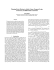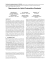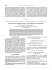# STAB52, An Introduction to Probability - Tutorial 8 Danny Cao, 996979845

## Transcription

STAB52, An Introduction to Probability - Tutorial 8 Danny Cao, 996979845
```STAB52, An Introduction to Probability - Tutorial 8
Danny Cao, 996979845
Department of Mathematics, University of Toronto
Consider the set of numbers x1 , ..., xn . Colloquially, the average of these values is understood to be
x
¯ = (x1 + ... + xn )/n. That is, we weight each value equally (probabilistically, every value xi is equally
likely
Pn to occur i.e. a discrete uniform distribution). However, what if each xi has probability pi of occurring
( i=1 pi = 1). Then if we average each value based on (weighting each value by) the probability of each
value occurring, then the average value becomes
n
X
pi · xi .
i=1
This is the motivation behind the expected value of a random variable: ”The average value that the random
variable takes weighted by the probabilities of each value”. More precisely, if X is discrete, then
X
X
E(X) =
x · P(X = x) =
x · P(X = x).
x∈R
x∈ supp(X)
Similarly, the expected value for an absolutely continuous random variable with density function fX (x) is
given by
Z ∞
E(X) =
xfx (x)dx.
−∞
Notice that this is the direct analog of the discrete case since an integral is essentially a way of summing
uncountably many values.
Problem 1. (Evans & Rosenthal, Exercise 3.1.16)
Suppose you start with one penny and repeatedly flip a fair coin. Each time you get heads, before the first
time you get tails, your number of pennies is doubled. Let X be the total number of pennies you have at the
end. Compute E(X).
Solution: Let Y be the number of heads flipped before the first tail so the Y ∼ geometric(1/2). This implies
P(Y = y) = 1/2y+1 , y = 0, 1, 2, .... It then follows that X = 2Y and so by the Theorem 3.1.1. (Evans &
Rosenthal), we have
E(X) = E(2Y ) =
X
2y P(Y = y) =
y
∞
X
2y ·
y=0
1
2y+1
=
∞
X
1
y=0
2
= ∞.
Notice here that we have an expected value of ∞; this not uncommon result.
Problem 2. (Expectation of a random variable with natural support)
Let X be a discrete random variable whose support is the natural numbers. Prove that
E(X) =
∞
X
P(X ≥ k)
k=1
.
Solution: Using the definition of expectation for discrete random variables, we rewrite as
E(X) =
∞
X
k · P(X = k) =
k=1
∞
X
k=1
1
P(X ≥ k)
Problem 3. (Evans & Rosenthal, Exercise 3.1.25.)
Suppose X is a discrete random variable, such that E(min(X, M )) = E(X). Prove that P(X > M ) = 0.
Solution: Allow me to first give you an incorrect solution. This is actually my first attempt at the problem
(and in fact, a more natural approach to this problem). Using the definition of expectation and the given
hypothesis, we compute
X
x · P(X = x) = E(X)
x∈R
= E(min(X, M ))
X
=
y · P(min(X, M ) = y)
y∈R
=
X
y · P(min(X, M ) = y) +
X
y · P(X = y) +
X
X
y · P(∅)
y>M
y≤M
=
y · P(min(X, M ) = y)
y>M
y≤M
=
X
y · P(X = y),
y≤M
P
which implies that y>M y · P(X = y) = 0. If we had the assumption that M was non-negative, we would
be done. However, this is not the case and we cannot actually conclude anything.
Now, let us see the correct solution: Define Y = X − min(X, M ) so then Y is a discrete random variable
such that Y ≥ 0. By the given assumption (along with linearity of expectation), we have
X
E(Y ) =
y · P(Y = y) = 0.
y≥0
Since we are summing over {y ≥ 0} and P(Y = y) ≥ 0, it must be the case that P(Y = y) = 0 for all y > 0.
Disjoint additivity then says P(Y > 0) = 0 i.e.
0 = P(X > min(X, M )) = P(X > M )
as required.
Problem 4. (Evans & Rosenthal, Exercise 3.1.17.a)
Let X ∼ Geometric(θ), and let Y = min(X, 100). Compute E(Y ).
Solution: We compute
E(Y ) =
∞
X
y · P(Y = y)
y=0
=
100
X
x · P(X = x) +
x=0
=θ
100
X
∞
X
100 · P(X = x)
x=101
x
x · (1 − θ) + 100θ
x=0
∞
X
(1 − θ)x
x=101
2
We compute the second summation as
∞
X
(1 − θ)x =
x=101
∞
X
(1 − θ)x −
x=0
100
X
(1 − θ)x
x=0
1 − (1 − θ)100
1
−
=
1 − (1 − θ)
θ
(1 − θ)101
=
.
θ
In the first summation, we let S =
P100
x · (1 − θ)x and hence
x=0
θS = S − (1 − θ)S
=S−
100
X
x · (1 − θ)x+1
x=0
=
100
X
x · (1 − θ)x −
=
(y − 1) · (1 − θ)y
y=1
x=0
100
X
101
X
(1 − θ)x
x=1
=
100
X
(1 − θ)x − (1 − θ)
x=0
=
1 − (1 − θ)101
− (1 − θ)
θ
I leave the simplification of the final expression as an elementary exercise.
3
```

### ECO383 Economics of Education In-Class Test – Sample Solutions ∗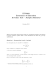### Math-UA.233.001: Theory of Probability Midterm cheatsheet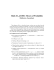### press kit - SIA Snow Show### press kit - SIA Snow Show### Price : 200 zł ROSENTHAL PORCELAIN Signed: CLASSIC ROSE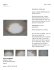### Price : 1 300 zł ROSENTHAL PORCELAIN Signed: CLASSIC ROSE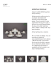### UNIT 2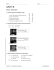### Decision Making Theory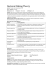### Math 335 Fall 2013, Exam 2 Key , , = 〈2 , + , −3 〉.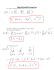### Florikan Case Study - Economic Development Council of Sarasota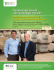### DS-eTPC Extrusion Touch Panel Control - Davis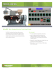### WS1800 - Westronic Systems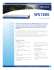### Bringing Compute to the Data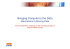### CRN 21389 Course Administration - UCO Department of Computer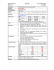### Uncertainty, Discretization and Saturation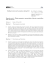### IEEE Paper Template in A4 (V1) - International Refereed Journal of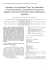### Jan Vondrák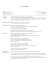### The brain-machine disanalogy revisited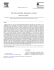### ECE 308 - Cal Poly Pomona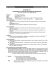### Formatting Instructions for Authors Using LaTeX